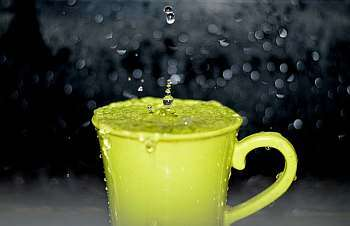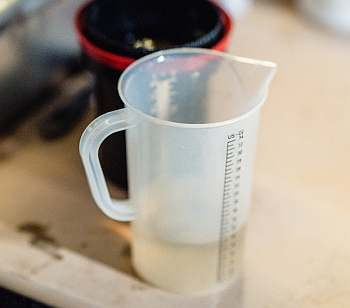# Cups to Quarts

Instructions: Use this calculator to convert cups to quarts, showing all the steps. Please type the number of cups you would like to convert in the form below:Type the number of cups:

## Using this Cups to Quarts Conversion

Cups and quarts are commonly used volume units used within the imperial unit system, and one of its characteristics is to have many different denominations for volume.

This variety of different units makes it difficult to memorize them, though those units are simply related by easy to memorize constants like 2, 4, 16, etc.

So, despite the fact that these conversions are algebraically simple, chances are that you won't remember them all, and you will need to use a conversion tool.## What is 1 quart in cups?

The basic question, to get started, is what is one quart in cups? Answer is simple: 1 quart is equivalent to 4 cups. And here we are thinking about standard US cups.

## How do you convert cups to quarts

This is done through proportions. You may remember that there is a conversion formula, but that formula is derived from a simple proportion, based on the basic equivalence 1 qt = 4 cups.

## What are the steps for the conversion of cups into quarts

1. The first step is to observe that 1 quart is equivalent to 4 cups. This is the point where your proportion is defined
2. Second, assume you are given C cups. You want to know many quarts (Q) will those C cups equivalent to
3. Third, the corresponding direct proportion that is needed is given by $$\displaystyle \frac{1}{4} = \frac{Q}{C}$$
4. Fourth, solving the proportion shown above for Q, we get the following conversion formula: $$Q = \displaystyle \frac{C}{4}$$

## Why would you want to convert cups to quarts

Different situations can require a conversion of units. Some products come labeled with one unit you are not familiar with, and you may want to know precisely the content based on a unit you are more familiar with.### Examples: Converting cups to quarts

Convert 4 cups to quarts

Solution: This is an easy one. The first step is to identify the amount we want to convert. In this case, we want to convert C = 4 cups. The corresponding conversion formula is

$Q = \displaystyle \frac{C}{4}$

The second step is to plug the corresponding value in, in this case C = 4 in the formula

$Q = \displaystyle \frac{C}{4} = Q = \displaystyle \frac{4}{4} = 1 \text{ qt}$

### Examples: More cups to quarts conversions

Convert 1 quart top cups

Solution: The conversion formula is:

$Q = \displaystyle \frac{C}{4}$

but in this case we are given Q = 1, instead of C, so we will solve for C:

$\Rightarrow C = 4Q$

So now, plugging Q = 1 in:

$C = 4 \times 1 = 4$

so the conclusion is that 1 quart is the same as 4 cups.

## Other volume conversions

There are other calculators of interest related to the conversion of cups to other units. For example, you can do a ounces to cups conversion using a similar proportionality equation.

Also, when dealing with different unit systems, you can use conversions like ounces to liters, and from liters to ounces.

Other very common one is the conversion of quarts to gallons.